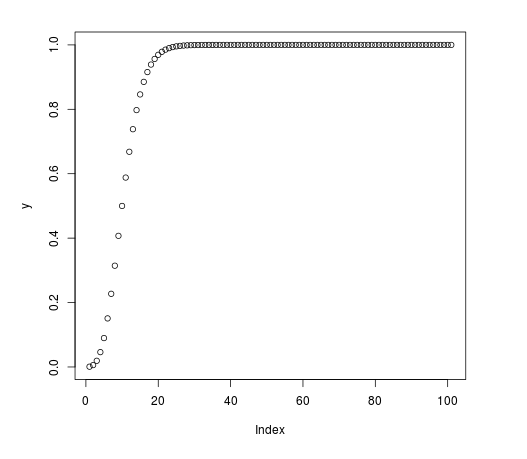# Compute the Negative Binomial Cumulative Density in R Programming – pnbinom() Function

`pnbinom()` function in R Language is used to compute the value of negative binomial cumulative density. It also creates a density plot of the negative binomial cumulative distribution.

Syntax: pnbinom(vec, size, prob)

Parameters:
vec: x-values for binomial density
size: Number of trials
prob: Probability

Example 1:

 `# R program to compute ` `# Negative Binomial cumulative Density ` ` `  `# Vector of x-values ` `x <``-` `seq(``0``, ``10``, by ``=` `1``) ` ` `  `# Calling pnbinom() Function ` `y <``-` `pnbinom(x, size ``=` `10``, prob ``=` `0.5``) ` `y `

Output:

```  0.0009765625 0.0058593750 0.0192871094 0.0461425781 0.0897827148
 0.1508789063 0.2272491455 0.3145294189 0.4072647095 0.5000000000
 0.5880985260
```

Example 2:

 `# R program to compute ` `# Negative Binomial Cumulative Density ` ` `  `# Vector of x-values ` `x <``-` `seq(``0``, ``100``, by ``=` `1``) ` ` `  `# Calling pnbinom() Function ` `y <``-` `pnbinom(x, size ``=` `10``, prob ``=` `0.5``) ` ` `  `# Plot a graph ` `plot(y) `

Output:My Personal Notes arrow_drop_upCheck out this Author's contributed articles.

If you like GeeksforGeeks and would like to contribute, you can also write an article using contribute.geeksforgeeks.org or mail your article to contribute@geeksforgeeks.org. See your article appearing on the GeeksforGeeks main page and help other Geeks.

Please Improve this article if you find anything incorrect by clicking on the "Improve Article" button below.

Article Tags :

Be the First to upvote.

Please write to us at contribute@geeksforgeeks.org to report any issue with the above content.